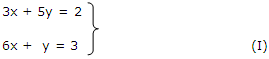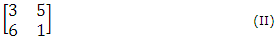# Explain the term matrix

Publish On: 2019-02-24

Total Post: 755

# Mjay Jollay

Total Post: 0

## ANS: Explain the term matrix

The student is already familiar with the system of simultaneous linear equations. For example, the equationis a system of two linear equations in two unknown (variables) x and y.

The left-hand side of (I) is completely known if we know the coefficients of various equations. We arrange these coefficients in a block as given below.In this arrangement, the entry [3, 5] in the top horizontal line is called the first row and it indicates the coefficients of x, y of the first equation 3x + 5y = 2, of the system (I). The entries [6, 1] in the second horizontal line is called the second row and it indicates the coefficients of x, y in the equation 6x + y = 3.